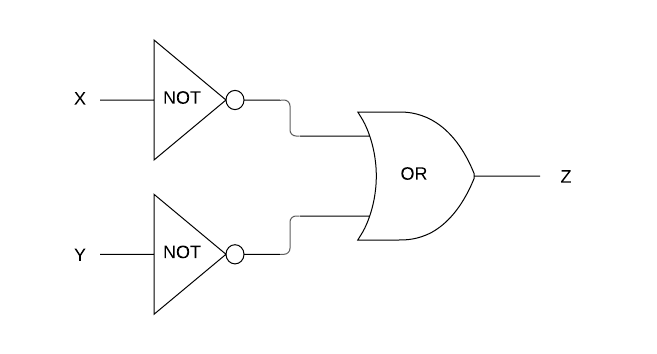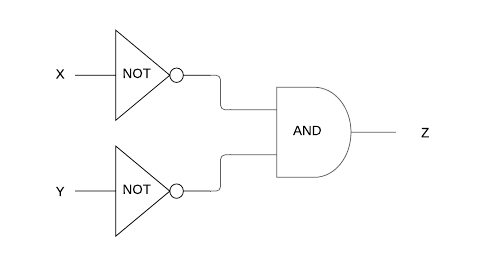Login or Create an Account to view the mark scheme, comment, and add to a test
(a).
Construct a truth table for the logic diagram represented below.(b).
What single gate is logically equivalent to the configuration represented in part (a)?

(c).
If, in part (a), the OR gate was replaced by an AND gate to produce the logic diagram below, what single gate would it then become logically equivalent to?HomeThe Right Test - Fast